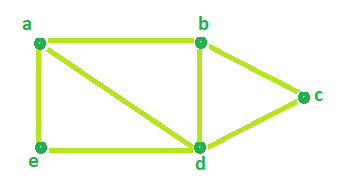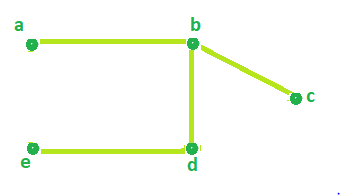# Program to find Circuit Rank of an Undirected Graph

Given the number of Vertices and the number of Edges of an Undirected Graph. The task is to determine the Circuit rank.

Circuit Rank: The Circuit rank of an undirected graph is defined as the minimum number of edges that must be removed from the graph to break all of its cycles, converting it into a tree or forest.

Examples

```Input :Edges = 7 , Vertices = 5
Output : Circuit rank = 3

Input : Edges = 7 , Vertices = 6
Output : Circuit rank = 2```

Formula

`Circuit rank = Edges - (Vertices - 1)`

Look at the sample graph below,Total number of Edges = 7 and Vertices = 5.
According to the above formula,

```Circuit Rank =  Edges - (Vertices - 1)
=  7 - (5 - 1)
= 3```

Therefore, Circuit Rank of the above graph = 3.
It can be seen in the below image that by removing 3 edges(a-d, a-e, c-d) from the above graph, all of it cycles can be removed.Implementation:

## C++

 `// C++ Program to find Circuit Rank of an Undirected Graph` `#include ` `using` `namespace` `std;`   `// Function that calculates the` `// Circuit rank of the Graph.` `int` `Rank(``int` `Edges, ``int` `Vertices)` `{` `    ``int` `result = 0;`   `    ``// calculates Circuit Rank` `    ``result = Edges - Vertices + 1;`   `    ``return` `result;` `}`   `// Driver Code` `int` `main()` `{` `    ``int` `Edges = 7, Vertices = 5;`   `    ``cout << ``"Circuit Rank = "` `<< Rank(Edges, Vertices);`   `    ``return` `0;` `}`

## Java

 `//Java Program to find Circuit Rank of an Undirected Graph`   `public` `class` `GFG {`   `    ``//Function that calculates the` `    ``//Circuit rank of the Graph.` `    ``static` `int` `Rank(``int` `Edges, ``int` `Vertices)` `    ``{` `     ``int` `result = ``0``;`   `     ``// calculates Circuit Rank` `     ``result = Edges - Vertices + ``1``;`   `     ``return` `result;` `    ``}`   `    ``//Driver Code` `    ``public` `static` `void` `main(String[] args) {` `        `  `         ``int` `Edges = ``7``, Vertices = ``5``;`   `         ``System.out.println(``"Circuit Rank = "` `+ Rank(Edges, Vertices));` `    ``}` `}`

## Python 3

 `# Python 3 program to find Circuit Rank of ` `# an Undirected Graph`   `# Function that calculates the ` `# Circuit rank of the Graph. ` `def` `Rank(Edges, Vertices) :`   `    ``# calculates Circuit Rank` `    ``result ``=` `Edges ``-` `Vertices ``+` `1`   `    ``return` `result`   `# Driver code     ` `if` `__name__ ``=``=` `"__main__"` `:`   `    ``Edges, Vertices ``=` `7``, ``5`   `    ``print``(``"Circuit Rank ="``,Rank(Edges, Vertices))`     `# This code is contributed by ANKITRAI1`

## C#

 `// C# Program to find Circuit` `// Rank of an Undirected Graph` `using` `System;`   `class` `GFG ` `{`   `// Function that calculates the` `// Circuit rank of the Graph.` `static` `int` `Rank(``int` `Edges,` `                ``int` `Vertices)` `{` `    ``int` `result = 0;` `    `  `    ``// calculates Circuit Rank` `    ``result = Edges - Vertices + 1;` `    `  `    ``return` `result;` `}`   `// Driver Code` `public` `static` `void` `Main()` `{` `    ``int` `Edges = 7, Vertices = 5;`   `    ``Console.WriteLine(``"Circuit Rank = "` `+ ` `                       ``Rank(Edges, Vertices));` `}` `}`   `// This code is contributed ` `// by inder_verma`

## PHP

 ``

## Javascript

 ``

Output

`Circuit Rank = 3`

Time Complexity: O(1)
Auxiliary Space: O(1)

Feeling lost in the world of random DSA topics, wasting time without progress? It's time for a change! Join our DSA course, where we'll guide you on an exciting journey to master DSA efficiently and on schedule.
Ready to dive in? Explore our Free Demo Content and join our DSA course, trusted by over 100,000 geeks!

Previous
Next